## Convective Operator

Defined for a Vector Fieldby, whereis the Gradient operator.

Applied in arbitrary orthogonal 3-D coordinates to a Vector Field B, the convective operator becomes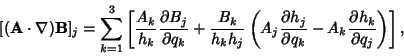(1)

where the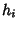s are related to the Metric Tensors by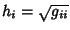. In Cartesian Coordinates,(2)
In Cylindrical Coordinates,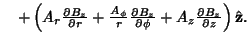(3)
In Spherical Coordinates,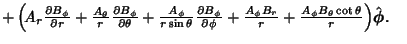(4)